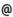## "Babes-Bolyai" University of Cluj-Napoca Faculty of Mathematics and Computer Science

 Mathematical modelling
 Code Semes-ter Hours: C+S+L Credits Type Section MME0003 4 2+2+0 6 compulsory Matematici aplicate
 Teaching Staff in Charge
 Lect. SERBAN Marcel Adrian, Ph.D., mserbanmath.ubbcluj.ro
 Lect. BUICA Adriana, Ph.D., abuicamath.ubbcluj.ro
 Aims -to present and solve some basic mathematical modells from various areas of science
 Content I. Mathematical modelling process 1. Introduction: Important steps in the mathematical history 2. Relation between mathematics and its applications 3. Mathematical modelling process 4. Simple mathematical models: a) Transports problem; b) Fibonacci's problem; c) Bank interest; d) Marketing expenses optimation II. Populations dynamics 1. Dynamics of an operator 2. Discrete dynamical systems 3. Stationary steady, stability 4. Autonomous systems 5. Mathematical models governed by differential equations a) Unlimited growth model b) Limited growth model c) Models with sacrifices d) Prey-predator models e) Mathematical models governed by differential equations with deviating argument f) SIR Epidemical models g) Periodicity phenomens in populations dynamics III. Mathematical models in sociology 1. Spiru Haret's models 2. Mario Bunge's models 3. Models for urbanization study 4. A probabilististical model for family size and structure prognoze IV. Spline functions 1. Spline functions efficient instrument in construction of optimal aproximation methods 2. Cubic spline functions, definition, construction 3. Cubic spline functions of interpolation 4. Convergence properties 5. Extremal convergence properties of cubic spline functions 6. Applications of spline functions in numerical treatment of equations 7. Histospline functions V. General diffusion models 1. Modern theory of diffusion 2. A general model diffusion-convection-reaction 3. Multispecies diffusion models
 References 1. Clements.R.R. Mathematical modelling,1989 2. Haret.S. Mecanica sociala.Ed.St.Bucuresti,1969 3. Iancu, C. Modelare matematica. Teme speciale. Ed. Casa cartii de stiinta, Cluj Napoca,2oo2 4. Murray,J.D.Mathematical biology, Springer-Verlag,Berlin,1989. 5. Yves Cherruault.Biomathematiques.Presses Univ. de France,1983 6. Rus.A.I.; C.Iancu.Modelare matematica. Ed. Transilvania Press, Cluj Napoca, 2000
 Assessment Exam.